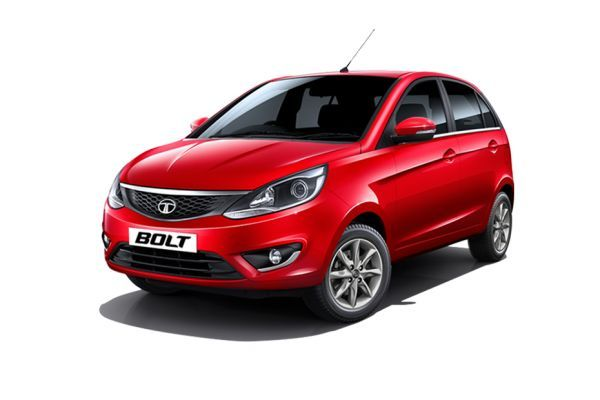×# Tata Bolt Service Cost

• Petrol
• Diesel

## Tata Bolt Maintenance cost

The estimated maintanence cost of Tata Bolt for 6 years is Rs. 21,304. The cost of first, second and third services are at Rs. 0, Rs. 2162 and Rs. 2802 respectively. The minimum distance travelled by Tata Bolt for 1st service is 4000 km.

## Tata Bolt Maintenance & Service Schedule

Total
1st Service
Rs. 0
2nd Service
Rs. 2162
3rd Service
Rs. 2802
4th Service
Rs. 3892
5th Service
Rs. 4805
6th Service
Rs. 3362
7th Service
Rs. 4282
Kms 4000 15000 30000 45000 60000 75000 90000
Month 3 12 24 36 48 60 72
Free/Paid Free Free Free Paid Paid Paid Paid
Part Change
Normal Engine Oil Rs. 0 Rs. 1080 Rs. 1080 Rs. 1080 Rs. 1080 Rs. 1080 Rs. 1080
Oil Filter Rs. 0 Rs. 200 Rs. 200 Rs. 200 Rs. 200 Rs. 200 Rs. 200
Air Filter Rs. 0 Rs. 270 Rs. 270 Rs. 270 Rs. 270 Rs. 270 Rs. 270
Coolant Rs. 0 Rs. 0 Rs. 0 Rs. 0 Rs. 803 Rs. 0 Rs. 0
Fuel Filter Rs. 0 Rs. 0 Rs. 400 Rs. 0 Rs. 400 Rs. 0 Rs. 400
Service Charge Rs. 0 Rs. 0 Rs. 0 Rs. 1200 Rs. 1200 Rs. 1200 Rs. 1200
Spark Plug Rs. 0 Rs. 0 Rs. 240 Rs. 0 Rs. 240 Rs. 0 Rs. 240
A.C.Filter Rs. 0 Rs. 0 Rs. 0 Rs. 250 Rs. 0 Rs. 0 Rs. 0
Transaxle Oil Rs. 0 Rs. 612 Rs. 612 Rs. 612 Rs. 612 Rs. 612 Rs. 612
Brake & Clutch Oil Rs. 0 Rs. 0 Rs. 0 Rs. 280 Rs. 0 Rs. 0 Rs. 280

Total estimated maintenance cost for 6 years

Rs. 21,304

### Tata Bolt OverviewTata Bolt Rs. 5.29 lakh